### Home > CALC > Chapter Ch2 > Lesson 2.2.1 > Problem2-51

2-51.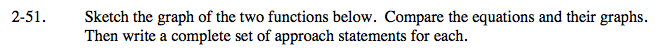For parts (a) and (b).
One of the functions has a hole and the other has an asymptote.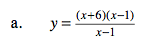Refer to Lesson 1.2.3, problems 1-44 & 1-45.

A complete set of approach statements include:

x → −∞
x → (hole)
x → (vertical asymptote)
x → ∞
x → (hole)+
x → (vertical asymptote)+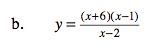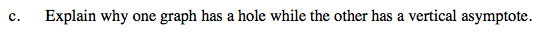How does algebra help determine the location of holes and vertical asymptotes?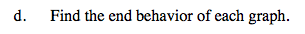Horizontal asymptotes, if they exist, are the same as end behavior. If there is no horizontal asymptote, find end behavior with polynomial division (ignore the remainder).